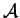Next: 4.2.2 Grid-, Model- and Up: 4.2 Input & Control Previous: 4.2 Input & Control

## 4.2.1 Global Definitions

To specify the simulation domain and the grid structure or distributed quantities it is possible to define a default input file that is used as a general input file in case no extra information is defined. In other words, if no further information is given by the user all instructions (grid-, geometry-, distributed quantity-, boundary initialization) read from this globally defined input file. If the user gets more specific in a subsection of the input file the global default is overwritten.

Source = PIF        # Information about the kind of source
# to be read.
{
Physical = <filename.pbf>
Logical  = <logical-name>
}


In the example above, a PIF-file [Fas91][Fas94] is assumed to be the global default input file if no other definition overwrites this default. The typical parameters which have to be defined to get access to various elements of a PIF-file include the name of the physical file as well as the logical name which holds information about geometry, grid and quantities.

A default output section can be defined very similar to the source structure that tells AMIGOS where to write its calculated results to. The syntax is nearly the same only the introducing keyword changes:

Output = PIF        # Information which output should be generated
{
Physical = <filename.pbf>
Logical  = <logical-name>
}


The third and last global structure is the definition of the time-step algorithm and its calculation. Its syntax looks like:

Time = <value>              # value is the total time in seconds that
# has to be calculated (end-time)
{
Step(<value>)            # defines the step size of the first time
# step
Epsilon(<value>)         # epsilon value describing the maximum
# error of the time discretization that
# is accepted during simulation
RejectionRatio(<value>)  # maximum multiplier how much smaller
# the time step should become in case
# of missing the epsilon criterion
StretchRatio(<value>)    # maximum multiplier how much bigger
# the next time-step can be in case
# of serving the epsilon condition
MaxNewton(<value>)       # maximum allowed Newton steps to solve
# the complete system
}


The time step is essential for some models to converge. If the user has chosen an initializing time step that is too big,MIGOS uses an automatic rejection mechanism that reduces the step size until convergence is achieved within the given user defined maximum number of Newton steps. This treatment has been chosen from empirical experiments stating that the total calculation time can often be reduced when simulating a single step by splitting it into two or even more sub-steps.Next: 4.2.2 Grid-, Model- and Up: 4.2 Input & Control Previous: 4.2 Input & Control